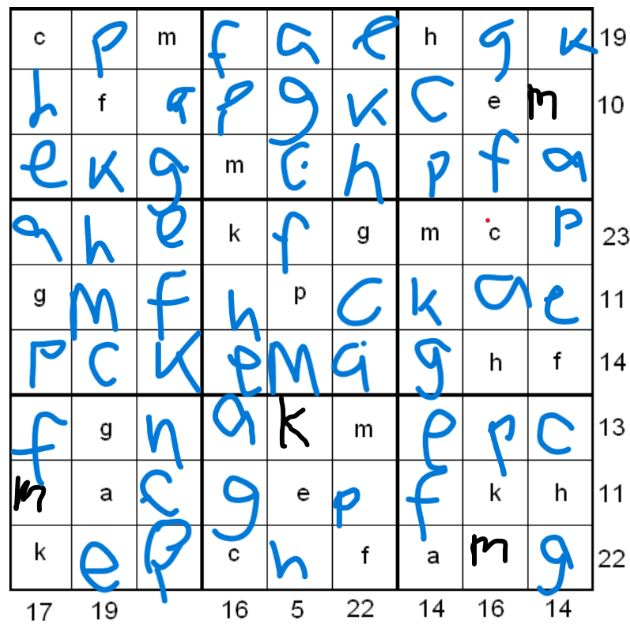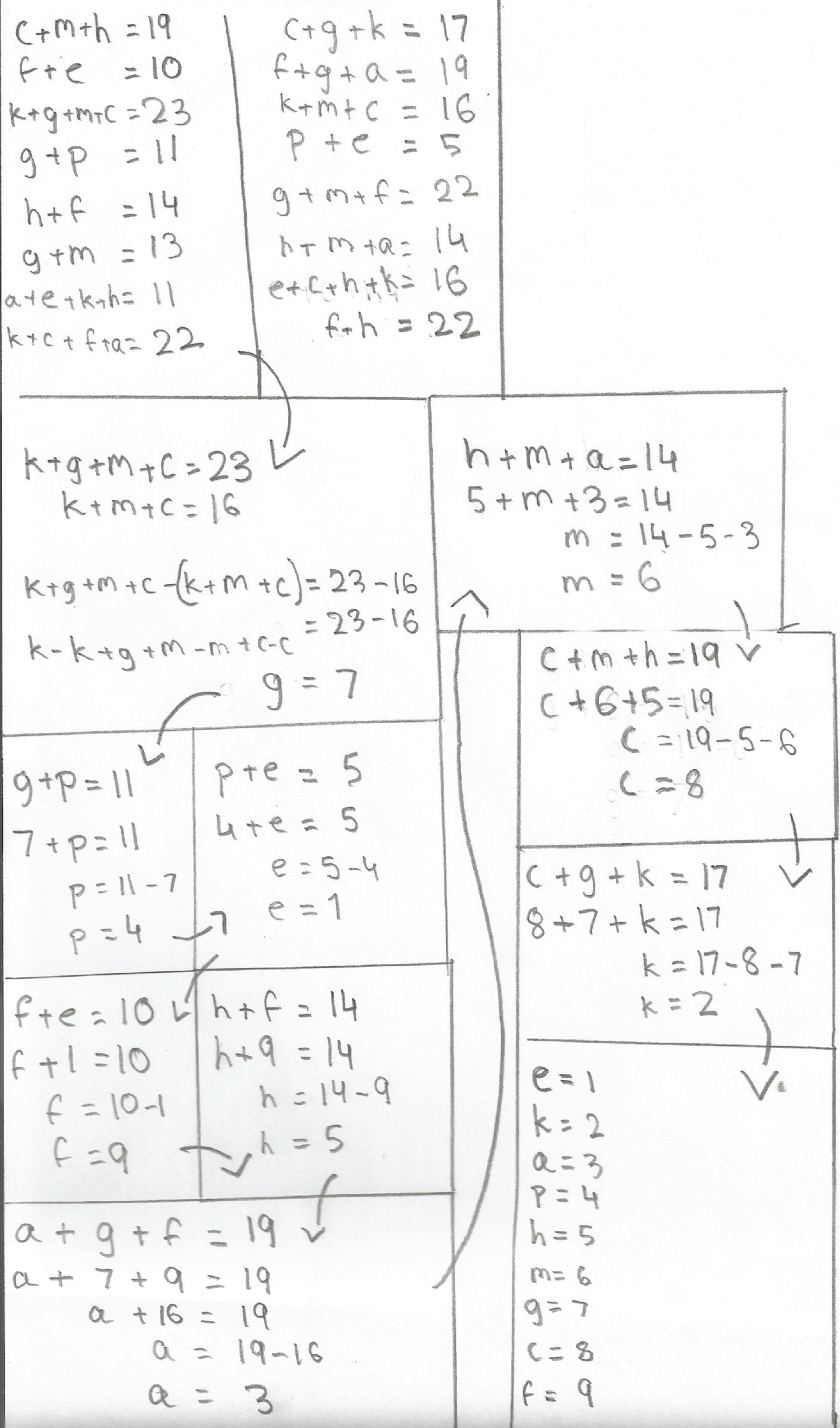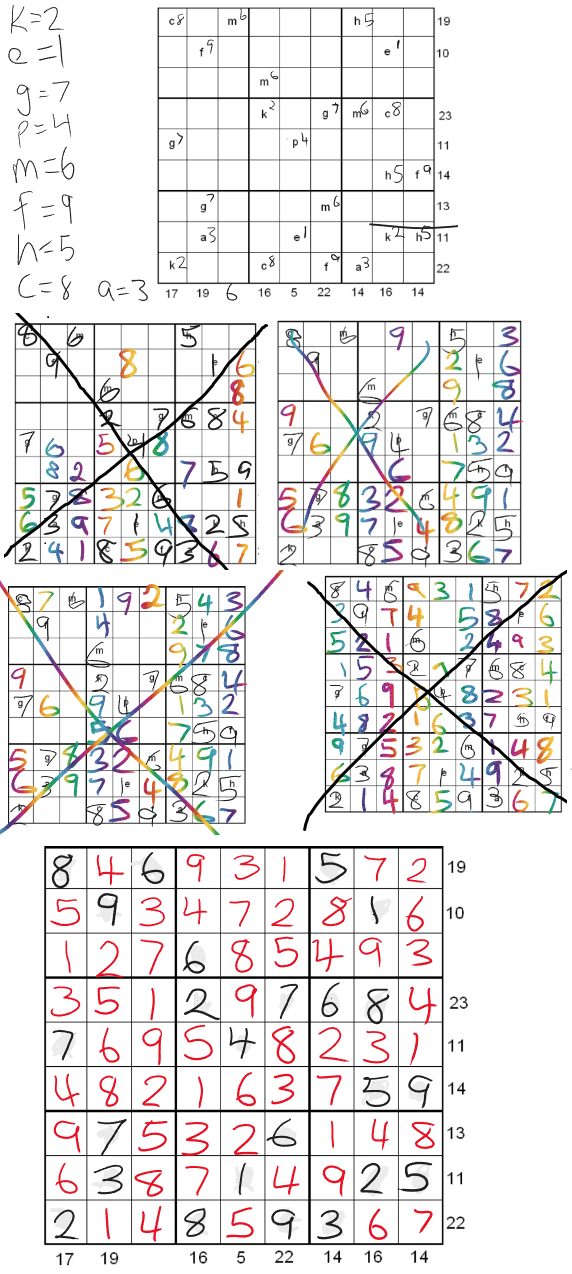#### You may also like### Real(ly) Numbers

If x, y and z are real numbers such that: x + y + z = 5 and xy + yz + zx = 3. What is the largest value that any of the numbers can have?### Overturning Fracsum

Can you solve the system of equations to find the values of x, y and z?### Building Tetrahedra

Can you make a tetrahedron whose faces all have the same perimeter?

# Simultaneous Equations Sudoku

##### Age 11 to 18Challenge Level

Some people approached this problem by first solving the simultaneous equations and then filling in the sudoku. Caleb, Megan and Tamsyn from Frederick Irwin Anglican School in Australia filled in the sudoku grid with letters and then solved the simultaneous equations to replace the letters with numbers. This is Megan and Tamsyn's work (click on the image to see a larger version):Caleb, Megan and Tamsyn didn't show their working for the simultaneous equations, but they correctly completed their sudoku grids.

Saanvi from Newcastle under Lyme Prep School in England, Neel from Zurich International school, Darren from Harrow School in the UK, Abhinav from St. Olaves Grammar School in England, Ci Hui Minh Ngoc Ong from Kelvin Grove State College (Brisbane) in Australia and Jiali showed how they solved the simultaneous equations.

Neel, Abhinav and Darren started from the fourth column and fourth row. This is Neel's work:Saanvi and Ci Hui Minh Ngoc Ong started from the first column and fourth row. This is Saavni's work:

Since the difference between $c+g+k$ and $k+g+m+c$ is $m,$ $m$ is $23-17$ which is $6.$
In the third row up, $g$ must be $7$ as $13-6=7.$
In the fifth row, $p$ must be $4$ since $11-7=4.$
In the sixth column, $f$ is $9$ since $22-13=9.$
In the second row, $e$ must be $1$ because $10-9=1.$
In the sixth row, $h$ is $5$ since $14-9=5.$
In the first row, $c$ is $8$ because $19-11=8.$
In the first column, $k$ must be $2$ as $17-15=2.$
By process of elimination, $a$ must be $3,$ but with this information you could easily work that out.

Jiali started from the sixth column and the seventh row. Jiali also suggested how to then fill in the sudoku:

1st Step: Find the values of the variables in the sudoku.
Since $g + m = 13$ and $g + m + f = 22,$ therefore $13 + f = 22$ and $f = 9.$
Since $f = 9$ and $f + h = 14,$ therefore $h = 5.$
Since $f = 9$ and $f + e = 10,$ therefore $e = 1.$
Since $e = 1$ and $p + e = 5,$ therefore $p = 4.$
Since $p = 4$ and $g + p = 11,$ therefore $g = 7.$
Since $f = 9, g = 7$ and $f + g + a = 19,$ therefore $a = 3.$
Since $g = 7$ and $g + m = 13,$ therefore $m = 6.$
Since $m = 6, h = 5$ and $c + m + h = 19,$ therefore $c = 8.$
Since $c = 8, g = 7$ and $c + g + k = 17,$ therefore $k = 2.$

2nd Step: Solve the sudoku.
After finding out what the variables represent, draw up a grid and write the numbers (that you have found) in the place of the variables. This should make it easier to solve the sudoku. Now there's the usual sudoku that you can solve. I solved mine by filling out all the 6's first, then gradually worked down the possibilities of where the numbers can go.

Indii A, Saskia B and Mignione K from Frederick Irwin Anglican School and Darren sent in partially completed sudokus to show their journeys to the correct solution. Here is Indii A, Saskia B and Mignione K's work, which shows a few wrong turns.